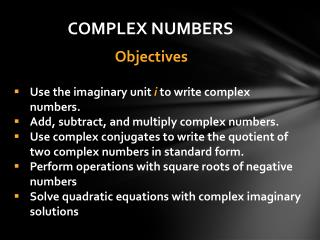DownloadDownload PresentationUse the imaginary unit i to write complex numbers. Add, subtract, and multiply complex numbers.

# Use the imaginary unit i to write complex numbers. Add, subtract, and multiply complex numbers.

Download Presentation## Use the imaginary unit i to write complex numbers. Add, subtract, and multiply complex numbers.

- - - - - - - - - - - - - - - - - - - - - - - - - - - E N D - - - - - - - - - - - - - - - - - - - - - - - - - - -
##### Presentation Transcript

1. COMPLEX NUMBERS Objectives • Use the imaginary unit i to write complex numbers. • Add, subtract, and multiply complex numbers. • Use complex conjugates to write the quotient of two complex numbers in standard form. • Perform operations with square roots of negative numbers • Solve quadratic equations with complex imaginary solutions

2. R Complex Numbers C Real Numbers R Irrational Numbers Q -bar Integers Z Imaginary Numbers i Whole numbers W Natural Numbers N Rational Numbers Q

3. What is an imaginary number? • It is a tool to solve an equation and was invented to solve quadratic equations of the form . • . • It has been used to solve equations for the last 200 years or so. • “Imaginary” is just a name, imaginary do indeed exist; they are numbers.

4. The Imaginary Unit i Previously, when we encountered square roots of negative numbers in solving equations, we would say “no real solution” or “not a real number”.

5. Complex Numbers & Imaginary Numbers a + bi represents the set of complex numbers, where aand bare real numbers and i is the imaginary part. a + bi is the standard form of a complex number. The real number a is written first, followed by a real number bmultiplied by i. The imaginary unit ialways follows the real number b, unless bis a radical. Example: If b is a radical, then write i before the radical.

6. Adding and Subtracting Complex Numbers (5 − 11i) + (7 + 4i) Simplify and treat the i like a variable. = 5 − 11i + 7 + 4i = (5 + 7) + (− 11i + 4i) = 12 − 7i Standard form

7. Adding and Subtracting Complex Numbers (− 5 +i) − (− 11− 6i) = − 5 + i + 11 + 6i = − 5 + 11 + i + 6i = 6 + 7i

8. (5 – 2i) + (3 + 3i)

9. (2 + 6i) − (12 − i)

10. Multiplying Complex Numbers 4i (3 − 5i) Standard form

11. Multiplying Complex Numbers (7 − 3i )( − 2 − 5i)use FOIL Standard form

12. 7i (2 − 9i) Standard form

13. (5 + 4i)(6 − 7i) • Standard form

14. Complex Conjugates • The complex conjugate of the number a + bi is a − bi. • Example: the complex conjugate of is • The complex conjugate of the number a − bi is a + bi. • Example: the complex conjugate of is

15. Complex Conjugates When we multiply the complex conjugates together, we get a real number. (a + bi) (a − bi) = a² + b² Example:

16. Complex Conjugates When we multiply the complex conjugates together, we get a real number. (a − bi) (a + bi) = a² + b² Example:

17. Using Complex Conjugates to Divide Complex Numbers Divide and express the result in standard form: 7 + 4i 2 − 5i The complex conjugate of the denominator is 2 + 5i. Multiply both the numerator and the denominator by the complex conjugate.

18. Divide and express the result in standard form: 5 + 4i 4 − i

19. Roots of Negative Numbers = • i = = 4 • i

20. Operations Involving Square Roots of Negative Numbers See examples on page 282.

21. The complex-number system is used to find zeros of functions that are not real numbers. When looking at a graph of a function, if the graph does notcross the x-axis, it has no real-number zeros.

22. A Quadratic Equation with Imaginary Solutions See example on page 283.